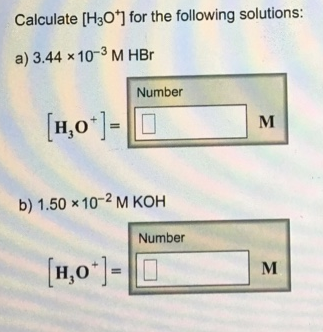Problem: Calculate [H3O+] for the following solutions:a. 3.44 × 10–3 M HBr[H3O+] = b. 1.50 × 10–2 M KOH[H3O+] =

FREE Expert Solution
85% (335 ratings)
FREE Expert Solution
85% (335 ratings)
Problem Details

Calculate [H3O+] for the following solutions:

a. 3.44 × 10–3 M HBr

[H3O+] =

b. 1.50 × 10–2 M KOH

[H3O+] =